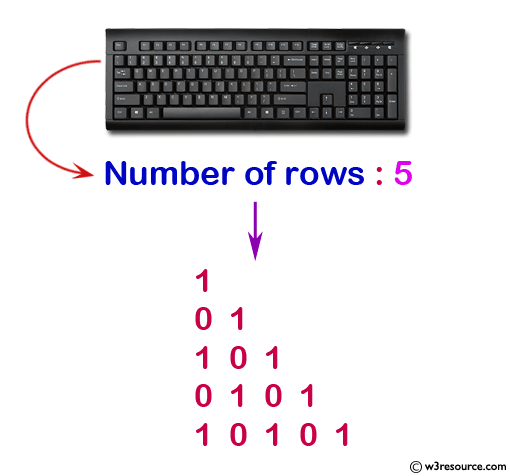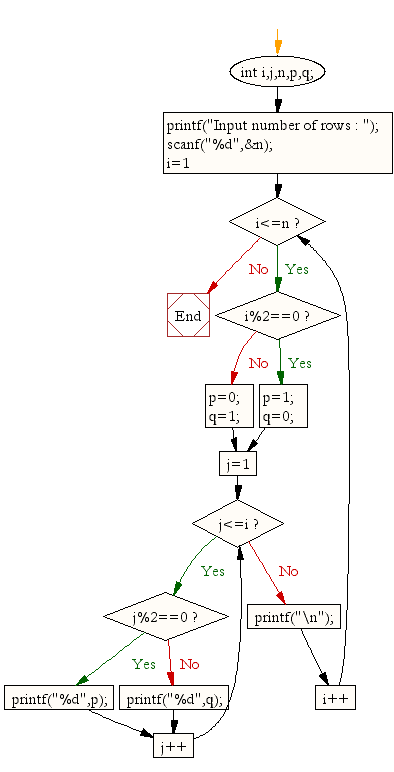﻿ C Program: Print the Floyd's Triangle - w3resource# C Exercises: Print the Floyd's Triangle

## C For Loop: Exercise-22 with Solution

Write a program in C to print the Floyd's Triangle. The Floyd's triangle is as below :

```1
01
101
0101
10101
```

Pictorial Presentation:Sample Solution:

C Code:

``````#include <stdio.h>

void main()
{
int i,j,n,p,q;
printf("Input number of rows : ");
scanf("%d",&n);
for(i=1;i<=n;i++)
{
if(i%2==0)
{ p=1;q=0;}
else
{ p=0;q=1;}
for(j=1;j<=i;j++)
if(j%2==0)
printf("%d",p);
else
printf("%d",q);
printf("\n");
}
}
```
```

Sample Output:

```Input number of rows : 5
1
01
101
0101
10101
```

Flowchart:C Programming Code Editor:

Improve this sample solution and post your code through Disqus.

What is the difficulty level of this exercise?

Test your Programming skills with w3resource's quiz.

﻿

## C Programming: Tips of the Day

You are seeing the ffffff because char is signed on your system. In C, vararg functions such as printf will promote all integers smaller than int to int. Since char is an integer (8-bit signed integer in your case), your chars are being promoted to int via sign-extension.

Since c0 and 80 have a leading 1-bit (and are negative as an 8-bit integer), they are being sign-extended while the others in your sample don't.

```char    int
c0 -> ffffffc0
80 -> ffffff80
61 -> 00000061
```
Here's a solution:
```char ch = 0xC0;
printf("%x", ch & 0xff);
```

This will mask out the upper bits and keep only the lower 8 bits that you want.

Ref : https://bit.ly/3vOLizM